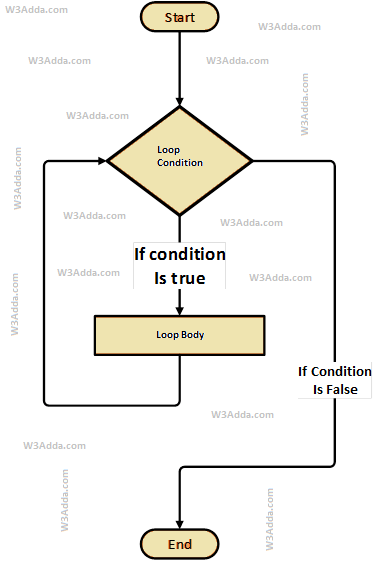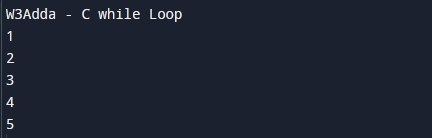# C while Loop

In this tutorial you will learn about the C while Loop and its application with practical example.

## C While Loop

The c while loop will execute a block of statements as long as a test expression is true. While loop is useful when the number of iterations can not be predicted beforehand. The while loop evaluates test expression at beginning of each pass.

The while loop is an entry-controlled loop. In a while loop, the test condition is evaluated before processing the loop body. If a condition is true then and only then the body of a loop is executed. Once the loop body is executed then control goes back to the beginning, and the condition is checked again if it is true, the same process is executed until the condition becomes false. Once the condition becomes false, the control goes out of the loop.

### C While Loop Flowchart

The following flowchart image illustrates the working of the while loop in C programming:### While Loop Syntax

Below is the general syntax of while loop in c programming:

Syntax:-

### Working Of While Loop

Here, while loop firstly evaluates the Condition inside parentheses `()`. The Condition is a Boolean expression that results in either True or False. If the condition results in True then statements inside the loop body are executed and the condition is evaluated again. This process is repeated until the condition is evaluated as False. If the condition results in False then execution is skipped from the loop body and the while loop is terminated.

After exiting the loop, the control goes to the statements immediately after the loop. The loop body can contain one or more statements. If it contains only one statement, then the curly braces are not compulsory. It is a good practice to use the curly braces even we have a single statement inside the loop body.

### While Loop Example

Below is a simple example to demonstrate the use of the while loop in c programming:

Example:-

Here, we have initialized ctr and maxCtr to 1 and 5 respectively, in the next statement we have a while loop, that checks the condition ctr <= maxCtr for each of the iterations. If the test condition returns True, the statements inside the loop body are executed and if the test condition returns False then the while loop is terminated. Inside the loop body, we have incremented the ctr value by one for each iteration. When we run the above C program, we see the following output –

Output:-### Properties of while loop

• A conditional expression is used to check the condition. The statements defined inside the while loop will repeatedly execute until the given condition fails.
• The condition will be true if it returns 0. The condition will be false if it returns any non-zero number.
• In a while loop, the condition expression is compulsory.
• Running a while loop without a body is possible.
• We can have more than one conditional expression in the while loop.
• If the loop body contains only one statement, then the braces are optional.

In this tutorial we have learn about the C while Loop and its application with practical example. I hope you will like this tutorial.